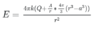# Electric field in a spherical shell

Eitan Levy
Homework Statement:
There is given a spherical shell with an inner radius of a and an outer radius of b, and there is a point charge +Q in the center. The volume charge density of the charge in the shell is A/r where A is some constant.

Find A if the electric field inside the shell is constant.
Relevant Equations:
Gauss' law
Hi, been a while since I last asked here something.

I am restudying electrostatics right now, and I am facing difficulties in the following question:

My attempt:

I tried to use Gauss' law, what I got is the equation in the capture but that doesn't lead me anywhere as I am unable to find a value that will result in a constant field inside the shell. What am I doing wrong?

Of course that the equation is for a<r<b

#### Attachments

•Capture.PNG
2 KB · Views: 148

Homework Helper
what I got
And how, precisely, did you get that ? (you want to know what you did wrong, so we want to know what you did. But all you telll us is the outcome -- which is wrong - or not)

My clues:
1. for QQ alone the field is ##kQ\over r^2##kQr2 and not 4π4π times as much
2. you multiply ##A\over r## with a volume -- that's not Gauss !

Eitan Levy
And how, precisely, did you get that ? (you want to know what you did wrong, so we want to know what you did. But all you telll us is the outcome -- which is wrong - or not)
Simply by looking at a sphere with a radius of r. The total charge inside the surface of this sphere should amount to Q, the point charge plus the charge in the shell until we reach a distance of r. Then I simply used the formula for Gauss' law.

Homework Helper
(I give up editing my post #2 - PF is whacking it all the time)

he charge in the shell until we reach a distance of r
How do you calculate that ?

Eitan Levy
(I give up editing my post #2 - PF is whacking it all the time)

How do you calculate that ?

A massive error by me, thank you.

Eitan Levy
(I give up editing my post #2 - PF is whacking it all the time)

How do you calculate that ?
Actually I am still unable to reach a solution.

I calculated that by calculating the integral of A/r*4πr^2dr from a to r, I got 2πA(r^2-a^2) as an answer.

EDIT: Never mind I got it, thanks.

Homework Helper
Gold Member
2022 Award
Homework Statement: There is given a spherical shell with an inner radius of a and an outer radius of b, and there is a point charge +Q in the center. The volume charge density of the charge in the shell is A/r where A is some constant.

Find A if the electric field inside the shell is constant.
You may be misinterpreting the question.
A spherically symmetric charge generates no field inside itself. They must mean the field is constant between the inner and outer radii of the shell.
This does not require integration. Consider a point at radius r between a and b. What is the field there due to:
- the point charge
- the spherical charge between a and r
- the spherical charge between r and b?

•collinsmark
Homework Helper
the spherical charge between a and r
requires integration ...

Homework Helper
Gold Member
requires integration ...Yes, that's true. (integration is required to find the charge inside the shell; or even the charge inside the shell from radius $a$ to $r$).

But I must be misinterpreting the problem. I can't seem to find a solution where both $E$ and $A$ are constants (for any $r$ that lies inside the spherical shell). I attribute that to my misunderstanding of something, somewhere or another. 🤔

Homework Helper
Gold Member
2022 Award
requires integration ...
It can be avoided. Consider the rate of change of the field as radius increases at radius a.
For the field due to the point charge that rate is ##-\frac{2Q}{a^3}##. For the spherical shell it is ##4\pi\frac Aaa^2\frac 1{a^2}=\frac{4\pi A}a##.
The sum of the two must be zero.
Arguably, this approach assumes there is an answer.

Last edited:
Homework Helper
Gold Member
But I must be misinterpreting the problem. I can't seem to find a solution where both $E$ and $A$ are constants (for any $r$ that lies inside the spherical shell). I attribute that to my misunderstanding of something, somewhere or another. 🤔
Nevermind. I see my misunderstanding now.For a specific value of A (depending on other paramters such as Q and a), both A and E can be made to be constants for the region between a and b.

Last edited: